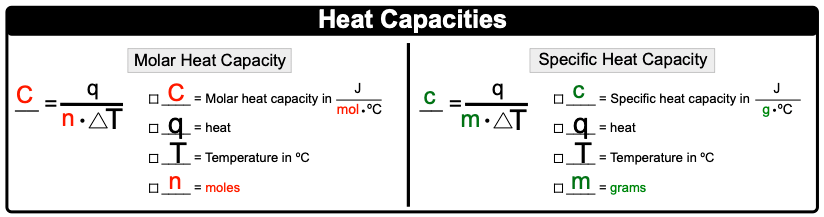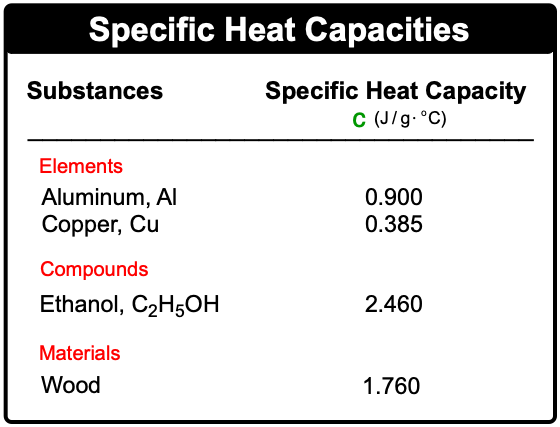Clutch Prep is now a part of Pearson
Ch.7 Energy, Rate and EquilibriumWorksheetSee all chapters

# Heat Capacity

See all sections
Sections
Nature of Energy
First Law of Thermodynamics
Endothermic & Exothermic Reactions
Bond Energy
Thermochemical Equations
Heat Capacity
Thermal Equilibrium (Simplified)
Hess's Law
Rate of Reaction
Energy Diagrams
Chemical Equilibrium
The Equilibrium Constant
Le Chatelier's Principle
Solubility Product Constant (Ksp)
Spontaneous Reaction
Entropy (Simplified)
Gibbs Free Energy (Simplified)

Heat Capacity is the amount of heat required to change the temperature of a substance.

###### Understanding Heat Capacity

Concept #1: Heat CapacityExample #1: If the temperature of a water bath goes from 25 K to 50 K, what can be said about the amount of heat?

Concept #2: Molar & Specific Heat CapacityExample #2: If 15.7 g of silver raises its temperature by 17.2 ºC when it absorbs 6845.5 J, what is its molar heat capacity?

Concept #3: Rearranged Specific Heat FormulaExample #3: How much heat (in kJ) is released when 120.0 g H2O goes from 90 ºC to 45 ºC? The specific heat capacity of H2O is 4.184 J / g  ºC.

Practice: A sample of copper absorbs 3.53 kJ of heat, which increases the temperature by 25 ºC, determine the mass (in kg) of the copper sample if the specific heat capacity of copper is 0.385 J / g ºC.

Practice: Based on their given specific heat capacities which compound would show the greatest temperature change upon absorbing 25.0 J of heat?Practice: 50.00 g of heated metal ore is placed into an insulated beaker containing 822.5 g of water. Once the metal heats up the final temperature of the water is 32.08 ºC. If the metal gains 14.55 kJ of energy, what is the initial temperature of the water? The specific heat capacity of copper is 4.184 J / g ºC.